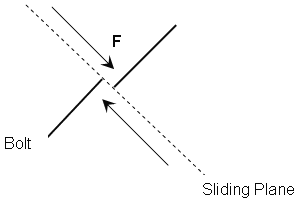The Rocscience International Conference 2021 Proceedings are now available. Read Now

Bolt Shear Strength

The Shear Strength of a bolt can be considered in the analysis, for any of the bolt types in UnWedge (except the Simple Bolt Force model).

To consider bolt shear strength:

1. Select the Use Shear Strength checkbox, for a bolt property type in the Bolt Properties dialog.
2. Enter a value of Shear Strength. The Shear Strength is simply entered as a force.

The shear strength of a bolt, refers to the shear capacity perpendicular to the axis of the bolt (i.e., what shear force would be required to shear through the cross-section of the bolt), as shown in the figure below.Shear force F required to fail bolt

Bolt Shear Strength Implementation

If bolt shear strength is considered, it will be implemented in the analysis as described in the Bolt Support Force topic in the Theory section.

• The shear force will only be applied if a bolt intersects a shearing (sliding) plane of a wedge. This corresponds to bolt failure modes D, E or F.
• Shear force will NOT be applied if a bolt intersects a dilating plane (failure modes A, B or C).
• If shear force is applied, the direction of the applied shear force will always be exactly opposite to the sliding direction of the wedge.

Bolt Efficiency and Shear Strength

Note that Use Bolt Orientation Efficiency is NOT used in conjunction with the Use Shear Strength option. If you have selected the Use Shear Strength option, and the bolt failure mode is D, E or F, so that the Bolt Shear Strength is applied, the Bolt Efficiency DOES NOT multiply the shear strength. The Bolt Efficiency is only used as a factor for the tensile bolt capacity determined from the Bolt Force Diagram. It has no effect on the Bolt Shear Strength.